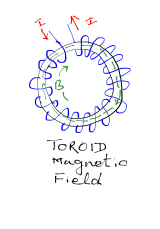## Wednesday, 18 May 2016

### Class 12 - Physics - Chapter 4: Moving Charges and Magnetism (VSQA)

Moving Charges and Magnetism

### Very Short Q & A

Q1: Define Magnetic Field.

Answer: The space around a magnet within which its effect is experienced is called Magnetic Field (B).

Q2: What is the SI unit of magnetic field?

Answer: Tesla. Another unit is Newton Ampere-1 metre-1

Q3: What is a solenoid?

Answer: A solenoid is a circular coil of a large number of turns, such that the turns of solenoid run parallel to its length. It acts similar to a bar magnet.

Q4: What is Lorentz force?

Answer: The total force on a charge (q) moving with a velocity (v) in the presence of magnetic and electric field (B and F) respectively is called Lorentz force.

Q5: What is a cyclotron?

Answer: It is a machine to accelerate charged particles or ions to high energies

Q6: What is the operational definition of one ampere?

Answer: One ampere is defined as that current flowing in each of two long parallel wires which are 1 metre apart resulting in a force of 2 x 10-7 N per metre of length of each wire.

Q7: What is the formula for cyclotron frequency?

Answer:  f = qB/(2Πm)

Q8: Define Biot-Savart Law.

Answer: The magnetic field (B) due to current element (dl) carrying a steady current I at a point P, at a distance r from the current element is:

B = (μ0/4π) I (dl x r)/r3

Q9: Which electric field or magnetic field can be used to stop a charged particle?

Answer: Electric field can be used to stop charged particle. Magnetic field can't be used since it is perpendicular and does not do any work.

Q10: What is a magnetic field due to a toroid?

Answer: Magnitude of magnetic field due to toroid is:

B = μ0nI

Q11: State the working principle of moving coil galvanometer.

Answer: It is based on the principle "when a current carrying coil id placed in a uniform magnetic field, the coil experiences a torque."

Q12: What is a toroid?

Answer: It is a solenoid bent into the form of closed region.

Q13: Is it possible to increase the range of a given voltmeter?

Answer: Yes. It can be done by connecting a resitance in series with voltmeter.

Q14: A proton and a deutron having equal momenta enter in a region of uniform magnetic field at right angle to the direction of the field. What would be their trajectories in the field.

Answer: Momenta and charge on both proton and deutron are same, the particle will follow a circular path of radius 1:1.#### 1 comment:

1.CBSE Board 10th Result 2016 will be published on 27th May 2016,